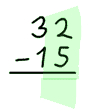# Quick Subtraction

This is a special method of subtraction
which many people find quickest (once they get used to it!).

## Start Normally

We start the same way as Subtraction by Regrouping (but we aren't going to be doing any regrouping):

• write down the larger number first and the smaller number directly below it
making sure to line up the columns!

• then do subtractions one column at a time like this (press play button):

## Smaller Number - Bigger NumberBut when a column has a smaller number on the top, do this: 1. Reverse the subtraction (to 5 − 2 = 3) 2. Find what makes it to 10 (3 + 7 = 10), then write that down as part of the answer 3. Add 1 to the digit on the left (of the number we are subtracting).

And this is how to do it:

With a bit of experience the "what adds to 10" becomes automatic. 1↔9, 2↔8, 3↔7, 4↔6, 5↔5, and the rest is easy. Practice at What Makes 10?.

Here is an example where we need to do it three times. This example is done at full speed (without showing all steps), so you may need to watch it a few times!